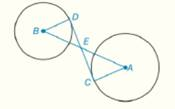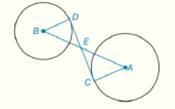Chapter 6.CR, Problem 27CR### Elementary Geometry for College St...

6th Edition
Daniel C. Alexander + 1 other
ISBN: 9781285195698

#### Solutions

Chapter
Section### Elementary Geometry for College St...

6th Edition
Daniel C. Alexander + 1 other
ISBN: 9781285195698
Textbook Problem
1 views

# In Review Exercises 27 to 29, give a proof for each statement. G i v e n :                     D C -   i s   t a n g e n t   t o   c i r c l e s   B   a n d   A   a t   p o i n t s   D   a n d   C ,   r e s p e c t i v e l y             P r o v e :                   A C · E D = C E · B DTo determine

To Prove: AC·ED=CE·BD, if DC- is tangent to circles B and A at points D and C, respectively.Explanation

Concept:

Theorem 6.2.3: The radius (or any other line through the center of a circle) drawn to a tangent at the point of tangency is perpendicular to the tangent at that point.

Calculation:

Given that DC- is tangent to circles B and A at points D and C, respectively.

By theorem 6.2.3, BDDE and ACCE.

So, BDE=ACE=90°

Because vertically opposite angles are equal, BED=AEC

### Still sussing out bartleby?

Check out a sample textbook solution.

See a sample solution

#### The Solution to Your Study Problems

Bartleby provides explanations to thousands of textbook problems written by our experts, many with advanced degrees!

Get Started

#### 7. Find the future value if \$8000 is invested for 10 years at compounded annually.

Mathematical Applications for the Management, Life, and Social Sciences

#### Find the median for the following set of scores: 1, 9, 3, 6, 4, 3, 11, 10

Essentials of Statistics for The Behavioral Sciences (MindTap Course List)

#### True or False: y = xex is a solution to y=y+yx.

Study Guide for Stewart's Single Variable Calculus: Early Transcendentals, 8th

#### 2 1 0 does not exist

Study Guide for Stewart's Multivariable Calculus, 8th